# PSAT Math : Slope and Line Equations

## Example Questions

← Previous 1 3

### Example Question #1 : Psat Mathematics

Based on the table below, when x = 5, y will equal

 x y -1 3 0 1 1 -1 2 -3

–10

11

–11

–9

–9

Explanation:

Use 2 points from the chart to find the equation of the line.

Example: (–1, 3) and (1, –1)

Using the formula for the slope, we find the slope to be –2.  Putting that into our equation for a line we get y = –2x + b.  Plug in one of the points for x and y into this equation in order to find b.  b = 1.

The equation then will be: y = –2x + 1.

Plug in 5 for x in order to find y.

y = –2(5) + 1

y = –9

### Example Question #1 : Geometry

What is the slope of a line that runs through points: (-2, 5) and (1, 7)?

3/2

2

2/3

5/7

2/3

Explanation:

The slope of a line is defined as a change in the y coordinates over a change in the x coordinates (rise over run).

To calculate the slope of a line, use the following formula: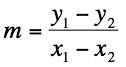### Example Question #1 : Geometry

A line passes through the points (–3, 5) and (2, 3). What is the slope of this line?

2/5

–2/3

-3/5

2/3

–2/5

–2/5

Explanation:

The slope of the line that passes these two points are simply ∆y/∆x = (3-5)/(2+3) = -2/5

### Example Question #1 : Slope And Line Equations

Which of the following lines intersects the y-axis at a thirty degree angle?

y = x√3 + 2

yx((√3)/3) + 1

y = x

y = x√2 - 2

y = x - √2

y = x√3 + 2

Explanation: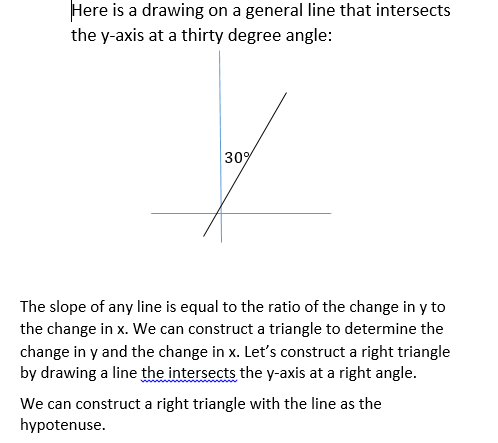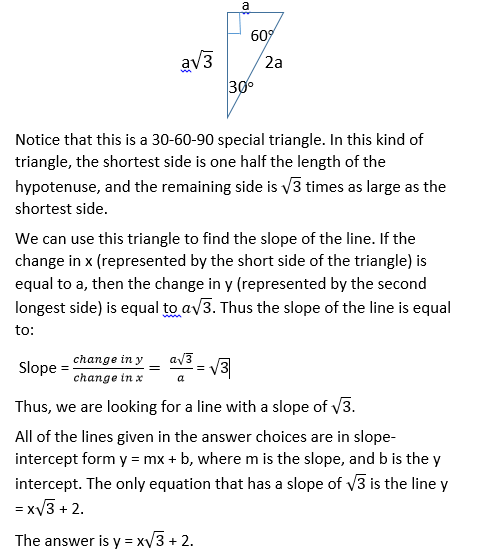### Example Question #19 : Other Lines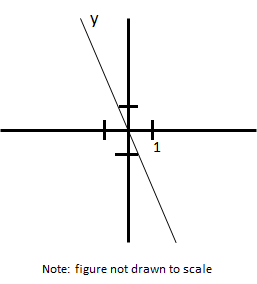What is a possible slope of line y?

–2

2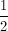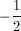–2

Explanation:

The slope is negative as it starts in quadrant 2 and ends in quadrant 4. Slope is equivlent to the change in y divided by the change in x. The change in y is greater than the change in x, which implies that the slope must be less than –1, leaving –2 as the only possible solution.

### Example Question #1 : Geometry

What is the slope between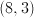and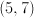?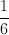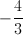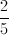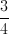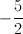Explanation:

Let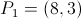and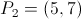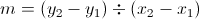so the slope becomes.

### Example Question #2 : Geometry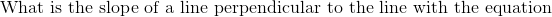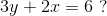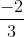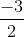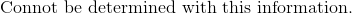Explanation: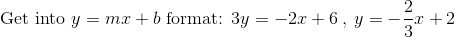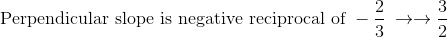### Example Question #1 : Geometry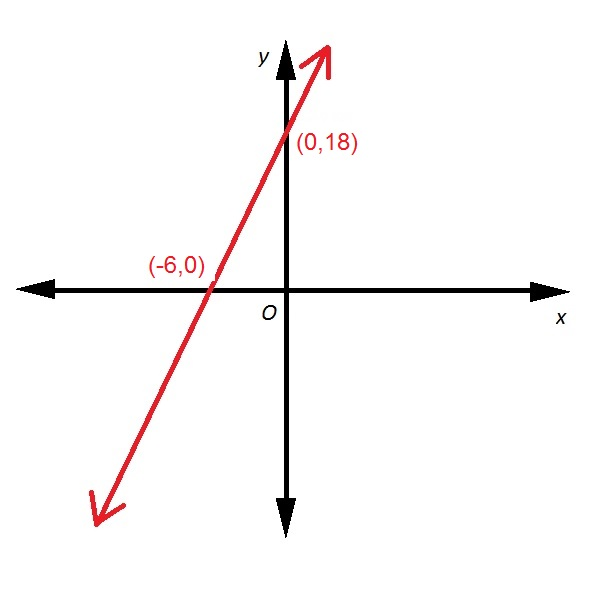Refer to above red line. What is its slope?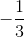Explanation:

The slope of a line. given two points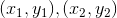can be calculated using the slope formula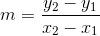Set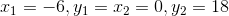: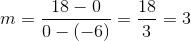### Example Question #3 : Geometry

Which of the following equations has as its graph a line with slope 4?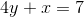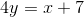None of the other responses is correct.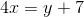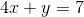Explanation:

For each equation, solve forand express in the slope-intercept form. The coefficient ofwill be the slope.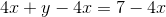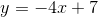Slope: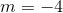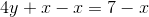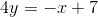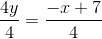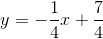Slope: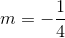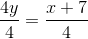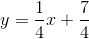Slope: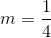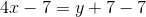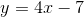Slope: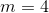The line of the equationis the one with slope 4.

### Example Question #1 : How To Find The Equation Of A Line

Solve the equation for x and y.

x – 4y = 245

5x + 2y = 150

= 545/9

y = –1375/18

x = 3

y = 7

= 234/5

= 1245/15

= –1375/9

= 545/18

= 545/9

y = –1375/18

Explanation:

While solving the problem requires the same method as the ones above, this is one is more complicated because of the more complex given equations. Start of by deriving a substitute for one of the unknowns. From the second equation we can derive y=75-(5x/2). Since 2y = 150 -5x, we divide both sides by two and find our substitution for y. Then we enter this into the first equation. We now have –x-4(75-(5x/2))=245. Distribute the 4. So we get –x – 300 + 10x = 245. So 9x =545, and x=545/9. Use this value for x and solve for y. The graph below illustrates the solution.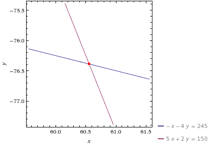← Previous 1 3

### All PSAT Math Resources